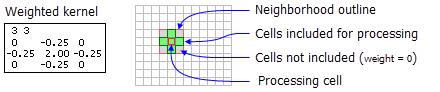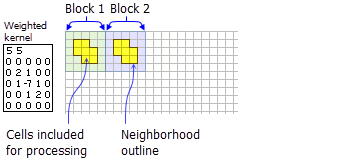# NbrWeight

Mit der Spatial Analyst-Lizenz verfügbar.

## Zusammenfassung

Defines a weight neighborhood which is created using a kernel file specifying the values to multiply the cell by that are within the neighborhood.

## AbbildungNbrWeight neighborhood example for the FocalStatistics function.NbrWeight neighborhood example for the BlockStatistics function.

## Auswertung

Tools that use neighborhood weight object: Block Statistics and Focal Statistics.

##### Lizenz:

This class is also available if you have an Image Analyst extension license, but only for the Focal Statistics tool.

The weight neighborhood is specified using a kernel file. The kernel file identifies which cell positions should be included within the neighborhood and the weights that will be used to multiply the cell values by on the input raster.

For focal tools the x,y position for the processing cell within the neighborhood, with respect to the upper-left corner of the neighborhood, is determined by the following equations:

`````` x = (width + 1)/2
y = (height + 1)/2``````

If the input number of cells is even, the x and y coordinates are computed using truncation.

The kernel file:

• The kernel file is an ASCII text file that defines the values and shape of a weight neighborhood.

• The first line specifies the width and height of the neighborhood (the number of cells in the x-direction, followed by a space, and the number of cells in the y-direction).

• The subsequent lines give the weight values of each position in the neighborhood. The values are input in the same configuration as appears in the neighborhood they represent. Positive, negative, and decimal values are all valid options to use as a weight. A space between each value is necessary.

• For locations in the neighborhood that are not to be part of the calculation, use a value of 0 at the corresponding location in the kernel file.

## Syntax

`NbrWeight (inKernelFile)`
 Parameter Erklärung Datentyp inKernelFile The inKernelFile is an ASCII text file that defines the shape of the neighborhood and the weight of each cell in that neighborhood. A value of 0 for a cell position indicates that the cell is not a member of the neighborhood, and a number at a corresponding cell's position indicates that the cell value be included as a member of the neighborhood. The nonzero value will also serve as the weight to multiply the corresponding cell value. File

## Eigenschaften

 Eigenschaft Erklärung Datentyp inKernelFile(Lesen und schreiben) The inKernelFile is an ASCII text file that defines the shape of the neighborhood and the weight of each cell in that neighborhood. A value of 0 for a cell position indicates that the cell is not a member of the neighborhood, and a number at a corresponding cell's position indicates that the cell value be included as a member of the neighborhood. The nonzero value will also serve as the weight to multiply the corresponding cell value. String

## Codebeispiel

NbrWeight example 1 (Python window)

Demonstrates how to create a NbrWeight class and use it in the BlockStatistics tool within the Python window.

``````import arcpy
from arcpy import env
from arcpy.sa import *
env.workspace = "C:/sapyexamples/data"
outNbrWF = BlockStatistics("block", NbrWeight("weight.txt"))
outNbrWF.save("C:/sapyexamples/output/blstatsnbrwf2")``````
NbrWeight example 2 (stand-alone script)

Implements the BlockStatistics tool using the NbrWeight class.

``````# Name: NbrWeight_Ex_02.py
# Description: Uses the NbrWeight object to execute BlockStatistics tool.
# Requirements: Spatial Analyst Extension

# Import system modules
import arcpy
from arcpy import env
from arcpy.sa import *

# Set environment settings
env.workspace = "C:/sapyexamples/data"

# Set local variables
inRaster = "block"

# Create the Neighborhood Object
inWeightFile = "C:/data/weight.txt"
myNbrWeight = NbrWeight(inWeightFile)

# Execute BlockStatistics
outBlStats =  BlockStatistics(inRaster, myNbrWeight, "MINIMUM", "DATA")

# Save the output
outBlStats.save("C:/sapyexamples/output/blstat_wght3")``````